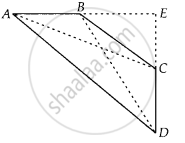# In a quadrilateral ABCD, ∠A + ∠D = 90°. Prove that AC2 + BD2 = AD2 + BC2 - Mathematics

Sum

In a quadrilateral ABCD, ∠A + ∠D = 90°. Prove that AC2 + BD2 = AD2 + BC2

#### Solution

Given: Quadrilateral ABCD, in which ∠A + ∠D = 90°

To prove: AC2 + BD2 = AD2 + BC2

Construct: Produce AB and CD to meet at E

Also join AC and BDProof: In ∆AED, ∠A + ∠D = 90° [given]

∴ ∠E = 180° – (∠A + ∠D) = 90°  ........[∵ sum of angles of a triangle = 180°]

Then, by Pythagoras theorem,

In ∆BEC, by Pythagoras theorem,

BC2 = BE2 + EC2

On adding both equations, we get

AD2 + BC2 = AE2 + DE2 + BE2 + CE2  ........(i)

In ∆AEC, by Pythagoras theorem,

AC2 = AE2 + CE2

and in ∆BED, by Pythagoras theorem,

BD2 = BE2 + DE2

On adding both equations, we get

AC2 + BD2 = AE2 + CE2 + BE2 + DE2  ........(ii)

From Eqs. (i) and (ii)

AC2 + BD2 = AD2 + BC2

Hence proved.

Concept: Application of Pythagoras Theorem in Acute Angle and Obtuse Angle
Is there an error in this question or solution?

#### APPEARS IN

NCERT Mathematics Exemplar Class 10
Chapter 6 Triangles
Exercise 6.4 | Q 12 | Page 74

Share